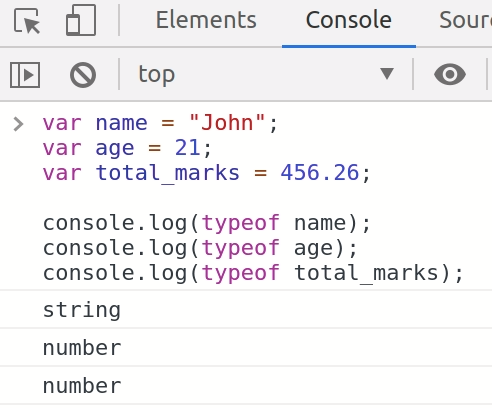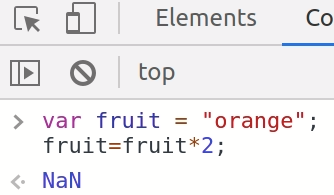Learn to Code via Tutorials on Repl.it!

← Back to all posts
Javascript Numbers

This post will cover topics related to assignment, condition and type checking of Numbers.

## Assigning integer and float value to a number.

``````var salary = 25000; //this is integer value
var average = 2.569; //this is float value ``````

## typeof Reserved Word

Using `typeof` is a reserved word that used to get the type of variable whether it may be integer, float, object, array and strings etc.

``````var name = "John";
var age = 21;
var total_marks = 456.26;

console.log(typeof name);
console.log(typeof age);
console.log(typeof total_marks);``````## NaN reserved word

NaN means Not a Number, these cannot be used to perform the mathematical operation.

``````var fruit = "orange";
fruit=fruit*2;``````By using with if conditions.

``````var fruit = "orange";
if(isNaN(fruit)){
console.log("This is not a number");
}else{
console.log("This is a number");
}

//Console Output
This is not a number``````

## Using `===` equal to operator

Using `===` will not only check if the value is equal but also check if is of equal type.

## using `==` equal to operator.

``````x = "10";
y = 10;
if(x==y){
console.log("Value of x is equal to y");
}else{
console.log("Value of x is not equal to y");
}

//Console Output
Value of x is equal to y``````

## using `===` equal to operator.

``````x = "10";
y = 10;
if(x===y){
console.log("Value of x is equal to y");
}else{
console.log("Value of x is not equal to y");
}

//Console Output
Value of x is not equal to y``````

You can find the original post here